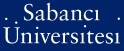# On the cycle structure of permutation polynomials

Çeşmelioğlu, Ayça (2008) On the cycle structure of permutation polynomials. [Thesis]Preview
PDF - Requires a PDF viewer such as GSview, Xpdf or Adobe Acrobat Reader
334Kb

## Abstract

L. Carlitz observed in 1953 that for any a € F*q, the transposition (0 a) can be represented by the polynomial Pa(x) = -a(((x - a)[q-2] + a-)[q-2] - a)[q-2] which shows that the group of permutation polynomials over Fq is generated by the linear polynomials ax + b, a, b € Fq, a≠0, and x[q-2]. Therefore any permutation polynomial over Fq can be represented as Pn = (...((ax + a)[q-2] +a) [q-2] ... + a[n])[q-2] + a[n+1], for some n ≥ 0. In this thesis we study the cycle structure of permutation polynomials Pn, and we count the permutations Pn, n ≤ 3, with a full cycle. We present some constructions of permutations of the form Pn with a full cycle for arbitrary n. These constructions are based on the so called binary symplectic matrices. The use of generalized Fibonacci sequences over Fq enables us to investigate a particular subgroup of Sq, the group of permutations on Fq. In the last chapter we present results on this special group of permutations.

Item Type: Thesis Finite fields. -- Permutation polynomials. -- Cycle structure. -- Sonlu cisimler. -- Permütasyon polinomları. -- Çevrim yapısı. -- Symmetric group on q letters. -- Monomials. -- Dickson polynomials. -- Cycle decomposition. -- Nonlinear pseudorandom number generators. Q Science > QA Mathematics 11345 IC-Cataloging 25 Feb 2009 15:36 25 Mar 2019 16:57

Repository Staff Only: item control page